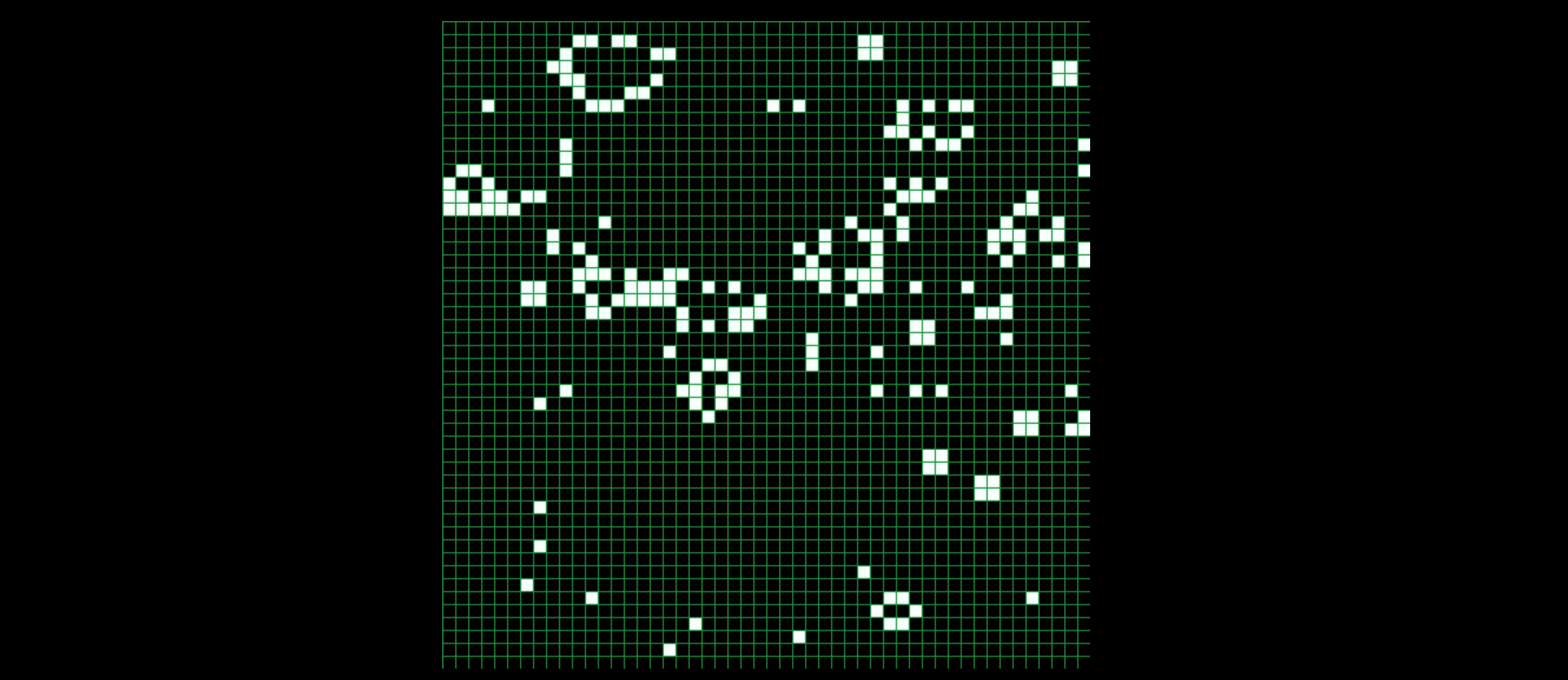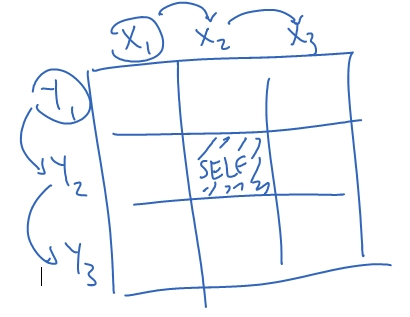# Game of Life

### Developer (JS App)## What is Game of Life?

“The ‘Game of Life’ is a zero-player game, meaning that its evolution is determined by its initial state. One interacts with the Game of Life by creating an initial configuration and observing how it evolves.”

Rules behind the Game of Life

The world in Game of Life is a 2D grid. Each square cell can be alive or dead, 1 or 0. There are 8 surrounding “neighbors” for each cell, top-left, top, top-right, left, right, bottom-left, bottom, and bottom-right. The rule is as follows:

1. A dead cell with exactly three live neighbors becomes live.
2. A live cell with exactly one live neighbor becomes dead.
3. A live cell with more than three live neighbors becomes dead.

If done correctly, a “glider” should occur as shown belowThe Plan

Our plan is to 1) Generate a random board and 2) Make the random life live and die according to the rules. I split creating the board into 3 steps. The first was to generate these “cells” that contained information like their location (x & y coordinates), their state and their future state (explained below). Second was to “draw” these cells to the board using their information. Lastly, the program would “count” the neighbors and update the information by going back to step 1 to “update” cell information and to step 2 to “draw” these steps out on the grid.The Problem

The idea is to draw these points on the grid, then under certain conditions chnage its state. It may sound simple at first, but the fact that we cannot change the state right away may make this program more confusing than it sounds.The plan I came up with is to, after creating a new class, to add a new parameter & instance variable. If we have it stored in the “next state” value, and made that separate from our “current state” value, the grid wouldn’t change and the actions would be accurate!

Life Class

The life class contains the simple variables of:

1. Position (X & Y)
2. Current State (Alive or Dead)
3. Next State (Alive or Dead)
``````int countNeighbor(int posX, int posY) {
int neighbor = 0;
for (int x = -1; x <= 1; x++) {
for (int y = -1; y <= 1; y++) {
if (y == 0 && x == 0) {
continue;
}
if ((posX + x >= 0 && posX + x < cols) && (posY + y >= 0 && posY + y < rows) && (myArray[posX+ x][posY + y].living == true)) {
neighbor++;
}
}
}
return neighbor;
}``````

Count ‘em Neighbors

We have to make a “countNeighbor” function that we can use to proccess (no pun intended) the information! We start by making a function with parameters of position X and Y, and initializing the a neighbor integer. Next, we’ll have to make a DOUBLE forloop that checks every single cell around the current point. Why DOUBLE? The first loop handles the X movement (from cell x1, x2, x3) and the second one handles the Y movement (repeating the X movement in y1, y2, y3). This effectively allows us to check x1y1, x2y1, x3y1, x1y2 and so on…Now that we can effectively check every cell, each cell needs to meet three conditions to be a “neighbor”. First of all, it has to be IN the grid, not outside and yield a “Out Of Bounds Exception”. So, 1) check is posX is within # of columns, 2) check if posY is within the # of rows. Lastly, we have to make sure that the cell is alive, so 3) cell.living == true

``````int countNeighbor(int posX, int posY) {
int neighbor = 0;
for (int x = -1; x <= 1; x++) {
for (int y = -1; y <= 1; y++) {
if (y == 0 && x == 0) {
continue;
}
if ((posX + x >= 0 && posX + x < cols) && (posY + y >= 0 && posY + y < rows) && (myArray[posX+ x][posY + y].living == true)) {
neighbor++;
}
}
}
return neighbor;
}``````

I have already initialized rows & cols previous to this code. Also, “continue” skips the loop. We want to do that because at y=0 and x=0 that is the given cell itself, which yields true (even though it’s not a neighbor)

The Core A.I.

### Generate a Random Board

First of all, I must create a board. This world is going to be a 2D grid, so I’ll be using a 2D array for that.

``````// Declare 2D array
Life[][] myArray = new Life[cols][rows];``````

Also, I would like the draw the points. We will get into drawing the points in the next section, but lets just pretend the drawLife function can draw life with parameters of drawLife(posX, posY, currentState). Creating a random board and drawing points both use double forloops, for we are placing values in a 2D array. The randBool function will be explained in the next section.

``````for (int i = 0; i < cols; i++) {
for (int j = 0; j < rows; j++) {
boolean status = randBool();

//the new life state can be true or false, doesn't matter
myArray[i][j] = new Life(i, j, status, false);
}
}

for (int i = 0; i < cols; i++) {
for (int j = 0; j < rows; j++) {
drawLife(i, j, myArray[i][j].living);
}
}``````

OH! Forgot to mention. We only want this happening once, so we must put all of this within a if statement that only runs once.

``````boolean start = true;

if (start == true) {
start = false;
//create a random board w/ code above
}``````

### Simulating "Life"

If you don’t remember the rules, here’s a refresher:

1. A dead cell with exactly 3 live neighbors becomes live.
2. A live cell with exactly 1 live neighbor becomes dead. .3 A live cell with more than 3 live neighbors becomes dead.

So the conditions, in coding language, is basically:

1. if (currentState == false && neighbors == 3) -> nextState = true
2. if (currentState == true && (neighbors == 1 || neighbors > 3) -> nextState = false Remember, we don’t want to intefere with the current grid, which is why we change the values of the next state. We then set all the values current values to the next values (after it’s all processed), and draw the life again.
``````myArray[i][j].living = myArray[i][j].next;
drawLife(i, j, myArray[i][j].living);
Java
The whole A.I. part of the code looks like this:

// create random board
if (start == true) {
start = false;
for (int i = 0; i < cols; i++) {
for (int j = 0; j < rows; j++) {
boolean status = randBool();
myArray[i][j] = new Life(i, j, status, false);
}
}

// Draw points
for (int i = 0; i < cols; i++) {
for (int j = 0; j < rows; j++) {
drawLife(i, j, myArray[i][j].living);
}
}
}

int neighbors;
// simulating life
for (int i = 0; i < cols; i++) {
for (int j = 0; j < rows; j++) {
neighbors = countNeighbor(i, j);
if (myArray[i][j].living == false && neighbors == 3) {
myArray[i][j].next = true;
}

if (myArray[i][j].living == true && (neighbors == 1 || neighbors > 3)) {
myArray[i][j].next = false;
}
}
}

//make the new values
for (int i = 0; i < cols; i++) {
for (int j = 0; j < rows; j++) {
myArray[i][j].living = myArray[i][j].next;
drawLife(i, j, myArray[i][j].living);
}
}``````

Finishing Touches

### Draw Life

I just created a function with x, y, state (alive or not) parameters to determine where to draw the life cell. I had a videoScale variable, which determined how “big” each cell was. If it was alive, I would fill in white (255), if not, I would fill in black (0). No matter the circumstance, I would still draw a border around each cell.

``````void drawLife (int posX, int posY, boolean life) {
int x = posX*videoScale;
int y = posY*videoScale;

//determine the cell color (filled in or not)
if (life) {
fill(255);
} else {
fill(0);
}

stroke(27, 155, 70); //draws border
rect(x, y, videoScale, videoScale); //fills in the actual cell
}``````

### Resetting the world

I didn’t want to restart the program everytime I wanted a new world with random values. I added a keyPress checker, if the user pressed “R”, then the board would reset (or start = true again and run it once more)!

``````if (keyPressed == true && (key == 'r' || key == 'R')) {
start = true;
}``````

### Setup & Variables

As I’ve explained along, there are some variables that were already initialized. Here are all of them:

``````//size of world
int cols = 50;
int rows = 50;

int videoScale = 10; //scale of the cells (10x10)

// Declare 2D array
Life[][] myArray = new Life[cols][rows];

boolean start = true;
Java
Because of our video scale of 10 and 50 columns & rows, the size of the window has to be 500x500 (50*10 = 500). Set the background to black and frameRate to 15. Increasing the frameRate would increase the speed of the simulation. I found 15 to be a good speed to view the simulation.

void setup() {
size(500, 500);
background(0);
frameRate(15);
}``````

Full Code + Demo

We’re DONE! Click here for the online demo. Links to download all the files have two options, Processing + Executable or just the Zipped .Pde file. Copy the full code for the lazy.